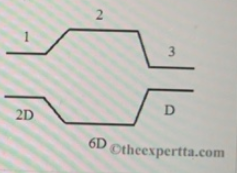# Problem: Water flows smoothly through a pipe with various circular cross- sections of diameters 2D, 6D, and D, respectively. Part (a) In section is the volume flow rate largest? Choose the best answer. A. Section 1B. Section 2C. Section 3D. They are the samePart (b) In which section is the speed of the water largest? Choose the best answer A. Section 1B. Section 2C. Section 3D. They are the samePart (c) What is the ratio of the speed in section 3 to the speed in section 1.Part (d) in which section is the pressure largest? Choose the best answer.A. Section 1B. Section 2C. Section 3D. They are the same

###### FREE Expert Solution

Flow rate:

$\overline{){\mathbf{Q}}{\mathbf{=}}{\mathbf{Av}}}$

Where Q is the flow rate, A is the are and v is the velocity.

Part (a)

The flow rate is constant.

83% (26 ratings)###### Problem Details

Water flows smoothly through a pipe with various circular cross- sections of diameters 2D, 6D, and D, respectively.Part (a) In section is the volume flow rate largest? Choose the best answer.

A. Section 1
B. Section 2
C. Section 3
D. They are the same

Part (b) In which section is the speed of the water largest? Choose the best answer

A. Section 1
B. Section 2
C. Section 3
D. They are the same

Part (c) What is the ratio of the speed in section 3 to the speed in section 1.

Part (d) in which section is the pressure largest? Choose the best answer.

A. Section 1
B. Section 2
C. Section 3
D. They are the same